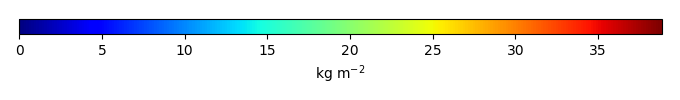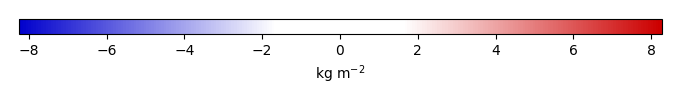# Mean State

Period Mean (original grids) [Pg]
Model Period Mean (intersection) [Pg]
Model Period Mean (complement) [Pg]
Benchmark Period Mean (intersection) [Pg]
Benchmark Period Mean (complement) [Pg]
Bias [kg m-2]
Bias Score 
Spatial Distribution Score 
Overall Score 
Benchmark [-] 32.8
CLM4 [-] 58.2 59.2 0.191 32.8 0.0526 6.64 0.474 0.642 0.558
CLM4.5 [-] 58.7 58.8 0.225 32.8 0.0526 6.61 0.397 0.530 0.463
CLM5 [-] 42.9 43.3 0.233 32.8 0.0526 2.86 0.650 0.863 0.757
Period Mean (original grids) [Pg]
Model Period Mean (intersection) [Pg]
Model Period Mean (complement) [Pg]
Benchmark Period Mean (intersection) [Pg]
Benchmark Period Mean (complement) [Pg]
Bias [kg m-2]
Bias Score 
Spatial Distribution Score 
Overall Score 
Benchmark [-] 8.35
CLM4 [-] 7.46 6.52 0.0693 8.34 0.0114 -0.305 0.606 0.881 0.744
CLM4.5 [-] 2.43 2.02 0.137 8.34 0.0114 -1.10 0.444 0.550 0.497
CLM5 [-] 3.54 2.95 0.191 8.34 0.0114 -0.934 0.497 0.662 0.580
Period Mean (original grids) [Pg]
Model Period Mean (intersection) [Pg]
Model Period Mean (complement) [Pg]
Benchmark Period Mean (intersection) [Pg]
Benchmark Period Mean (complement) [Pg]
Bias [kg m-2]
Bias Score 
Spatial Distribution Score 
Overall Score 
Benchmark [-] 10.6
CLM4 [-] 13.7 13.2 0.225 10.6 0.0246 0.363 0.496 0.488 0.492
CLM4.5 [-] 6.81 6.40 0.250 10.6 0.0246 -0.363 0.479 0.622 0.550
CLM5 [-] 17.1 16.4 0.388 10.6 0.0246 0.803 0.514 0.515 0.514
Period Mean (original grids) [Pg]
Model Period Mean (intersection) [Pg]
Model Period Mean (complement) [Pg]
Benchmark Period Mean (intersection) [Pg]
Benchmark Period Mean (complement) [Pg]
Bias [kg m-2]
Bias Score 
Spatial Distribution Score 
Overall Score 
Benchmark [-] 4.69
CLM4 [-] 0.756 0.756 0.0220 4.67 0.0241 -1.13 0.435 0.277 0.356
CLM4.5 [-] 3.26 3.23 0.0402 4.67 0.0241 -0.357 0.599 0.685 0.642
CLM5 [-] 5.06 5.01 0.0720 4.67 0.0241 0.237 0.599 0.858 0.728
Period Mean (original grids) [Pg]
Model Period Mean (intersection) [Pg]
Model Period Mean (complement) [Pg]
Benchmark Period Mean (intersection) [Pg]
Benchmark Period Mean (complement) [Pg]
Bias [kg m-2]
Bias Score 
Spatial Distribution Score 
Overall Score 
Benchmark [-] 27.8
CLM4 [-] 79.6 76.4 2.85 26.8 0.921 22.3 0.193 0.535 0.364
CLM4.5 [-] 66.8 64.1 2.46 26.8 0.921 17.8 0.275 0.784 0.530
CLM5 [-] 49.4 47.3 1.89 26.8 0.921 11.4 0.466 0.913 0.690
Period Mean (original grids) [Pg]
Model Period Mean (intersection) [Pg]
Model Period Mean (complement) [Pg]
Benchmark Period Mean (intersection) [Pg]
Benchmark Period Mean (complement) [Pg]
Bias [kg m-2]
Bias Score 
Spatial Distribution Score 
Overall Score 
Benchmark [-] 43.5
CLM4 [-] 38.4 37.5 0.157 43.5 0.0288 -0.536 0.579 0.794 0.687
CLM4.5 [-] 42.8 41.8 0.204 43.5 0.0288 -0.0592 0.545 0.763 0.654
CLM5 [-] 73.2 71.7 0.369 43.5 0.0288 3.33 0.501 0.547 0.524
Period Mean (original grids) [Pg]
Model Period Mean (intersection) [Pg]
Model Period Mean (complement) [Pg]
Benchmark Period Mean (intersection) [Pg]
Benchmark Period Mean (complement) [Pg]
Bias [kg m-2]
Bias Score 
Spatial Distribution Score 
Overall Score 
Benchmark [-] 32.0
CLM4 [-] 45.4 45.4 0.720 31.9 0.0804 1.17 0.522 0.682 0.602
CLM4.5 [-] 44.2 44.1 0.725 31.9 0.0804 1.09 0.458 0.662 0.560
CLM5 [-] 36.1 36.1 0.765 31.9 0.0804 0.598 0.504 0.751 0.628
Period Mean (original grids) [Pg]
Model Period Mean (intersection) [Pg]
Model Period Mean (complement) [Pg]
Benchmark Period Mean (intersection) [Pg]
Benchmark Period Mean (complement) [Pg]
Bias [kg m-2]
Bias Score 
Spatial Distribution Score 
Overall Score 
Benchmark [-] 21.1
CLM4 [-] 30.3 28.8 0.611 20.9 0.146 2.23 0.536 0.656 0.596
CLM4.5 [-] 25.6 24.1 0.649 20.9 0.146 1.66 0.455 0.566 0.511
CLM5 [-] 25.4 24.1 0.687 20.9 0.146 1.53 0.535 0.742 0.638
Period Mean (original grids) [Pg]
Model Period Mean (intersection) [Pg]
Model Period Mean (complement) [Pg]
Benchmark Period Mean (intersection) [Pg]
Benchmark Period Mean (complement) [Pg]
Bias [kg m-2]
Bias Score 
Spatial Distribution Score 
Overall Score 
Benchmark [-] 3.81
CLM4 [-] 0.856 0.941 0.0203 3.81 0.000319 -0.812 0.456 0.373 0.415
CLM4.5 [-] 3.54 3.69 0.0538 3.81 0.000319 -0.0140 0.616 0.835 0.725
CLM5 [-] 8.81 9.19 0.106 3.81 0.000319 1.63 0.458 0.675 0.566
Period Mean (original grids) [Pg]
Model Period Mean (intersection) [Pg]
Model Period Mean (complement) [Pg]
Benchmark Period Mean (intersection) [Pg]
Benchmark Period Mean (complement) [Pg]
Bias [kg m-2]
Bias Score 
Spatial Distribution Score 
Overall Score 
Benchmark [-] 9.16
CLM4 [-] 7.53 6.88 0.523 8.87 0.289 0.216 0.543 0.774 0.659
CLM4.5 [-] 7.58 6.88 0.581 8.87 0.289 0.219 0.575 0.829 0.702
CLM5 [-] 11.5 10.5 0.819 8.87 0.289 0.811 0.580 0.783 0.681
Period Mean (original grids) [Pg]
Model Period Mean (intersection) [Pg]
Model Period Mean (complement) [Pg]
Benchmark Period Mean (intersection) [Pg]
Benchmark Period Mean (complement) [Pg]
Bias [kg m-2]
Bias Score 
Spatial Distribution Score 
Overall Score 
Benchmark [-] 12.0
CLM4 [-] 8.10 7.78 0.185 12.0 0.0529 -0.409 0.585 0.828 0.706
CLM4.5 [-] 14.6 14.0 0.322 12.0 0.0529 0.861 0.621 0.770 0.695
CLM5 [-] 26.8 25.7 0.646 12.0 0.0529 3.42 0.443 0.261 0.352
Period Mean (original grids) [Pg]
Model Period Mean (intersection) [Pg]
Model Period Mean (complement) [Pg]
Benchmark Period Mean (intersection) [Pg]
Benchmark Period Mean (complement) [Pg]
Bias [kg m-2]
Bias Score 
Spatial Distribution Score 
Overall Score 
Benchmark [-] 368.
CLM4 [-] 582. 573. 9.02 363. 4.64 2.29 0.460 0.493 0.477
CLM4.5 [-] 560. 550. 9.81 363. 4.64 2.12 0.452 0.578 0.515
CLM5 [-] 589. 578. 11.2 363. 4.64 2.37 0.514 0.680 0.597
Period Mean (original grids) [Pg]
Model Period Mean (intersection) [Pg]
Model Period Mean (complement) [Pg]
Benchmark Period Mean (intersection) [Pg]
Benchmark Period Mean (complement) [Pg]
Bias [kg m-2]
Bias Score 
Spatial Distribution Score 
Overall Score 
Benchmark [-] 1.24
CLM4 [-] 1.35 1.20 0.140 1.23 0.00783 0.0345 0.555 0.809 0.682
CLM4.5 [-] 1.53 1.28 0.255 1.23 0.00783 0.0560 0.522 0.680 0.601
CLM5 [-] 1.68 1.27 0.401 1.23 0.00783 0.0633 0.494 0.673 0.584
Period Mean (original grids) [Pg]
Model Period Mean (intersection) [Pg]
Model Period Mean (complement) [Pg]
Benchmark Period Mean (intersection) [Pg]
Benchmark Period Mean (complement) [Pg]
Bias [kg m-2]
Bias Score 
Spatial Distribution Score 
Overall Score 
Benchmark [-] 5.62
CLM4 [-] 4.27 4.16 0.0382 5.59 0.0265 -0.181 0.571 0.828 0.699
CLM4.5 [-] 9.20 8.91 0.148 5.59 0.0265 0.659 0.536 0.796 0.666
CLM5 [-] 16.4 16.0 0.288 5.59 0.0265 1.94 0.297 0.357 0.327
Period Mean (original grids) [Pg]
Model Period Mean (intersection) [Pg]
Model Period Mean (complement) [Pg]
Benchmark Period Mean (intersection) [Pg]
Benchmark Period Mean (complement) [Pg]
Bias [kg m-2]
Bias Score 
Spatial Distribution Score 
Overall Score 
Benchmark [-] 8.21
CLM4 [-] 5.39 4.80 0.286 8.09 0.122 -0.207 0.461 0.424 0.443
CLM4.5 [-] 11.4 10.7 0.449 8.09 0.122 2.16 0.435 0.400 0.418
CLM5 [-] 11.9 11.1 0.518 8.09 0.122 2.42 0.498 0.495 0.497
Period Mean (original grids) [Pg]
Model Period Mean (intersection) [Pg]
Model Period Mean (complement) [Pg]
Benchmark Period Mean (intersection) [Pg]
Benchmark Period Mean (complement) [Pg]
Bias [kg m-2]
Bias Score 
Spatial Distribution Score 
Overall Score 
Benchmark [-] 14.8
CLM4 [-] 16.4 16.1 0.184 14.8 0.0147 0.622 0.658 0.786 0.722
CLM4.5 [-] 20.4 20.0 0.238 14.8 0.0147 1.63 0.607 0.670 0.639
CLM5 [-] 17.8 17.4 0.231 14.8 0.0147 1.04 0.629 0.717 0.673
Period Mean (original grids) [Pg]
Model Period Mean (intersection) [Pg]
Model Period Mean (complement) [Pg]
Benchmark Period Mean (intersection) [Pg]
Benchmark Period Mean (complement) [Pg]
Bias [kg m-2]
Bias Score 
Spatial Distribution Score 
Overall Score 
Benchmark [-] 20.1
CLM4 [-] 25.1 25.1 0.185 19.8 0.219 1.29 0.528 0.792 0.660
CLM4.5 [-] 34.8 34.8 0.196 19.8 0.219 3.16 0.488 0.793 0.641
CLM5 [-] 56.8 56.7 0.307 19.8 0.219 7.50 0.303 0.635 0.469
Period Mean (original grids) [Pg]
Model Period Mean (intersection) [Pg]
Model Period Mean (complement) [Pg]
Benchmark Period Mean (intersection) [Pg]
Benchmark Period Mean (complement) [Pg]
Bias [kg m-2]
Bias Score 
Spatial Distribution Score 
Overall Score 
Benchmark [-] 8.04
CLM4 [-] 5.11 5.13 0.0220 7.99 0.0515 -0.613 0.556 0.499 0.527
CLM4.5 [-] 3.42 3.43 0.0314 7.99 0.0515 -1.03 0.494 0.320 0.407
CLM5 [-] 3.49 3.46 0.0449 7.99 0.0515 -0.996 0.524 0.654 0.589
Period Mean (original grids) [Pg]
Model Period Mean (intersection) [Pg]
Model Period Mean (complement) [Pg]
Benchmark Period Mean (intersection) [Pg]
Benchmark Period Mean (complement) [Pg]
Bias [kg m-2]
Bias Score 
Spatial Distribution Score 
Overall Score 
Benchmark [-] 88.9
CLM4 [-] 209. 208. 0.419 88.8 0.0655 14.5 0.344 0.439 0.392
CLM4.5 [-] 181. 180. 0.460 88.8 0.0655 11.3 0.387 0.617 0.502
CLM5 [-] 152. 151. 0.431 88.8 0.0655 7.77 0.546 0.889 0.718
Period Mean (original grids) [Pg]
Model Period Mean (intersection) [Pg]
Model Period Mean (complement) [Pg]
Benchmark Period Mean (intersection) [Pg]
Benchmark Period Mean (complement) [Pg]
Bias [kg m-2]
Bias Score 
Spatial Distribution Score 
Overall Score 
Benchmark [-] 9.09
CLM4 [-] 20.1 20.3 0.120 9.08 0.0128 1.88 0.367 0.194 0.281
CLM4.5 [-] 15.5 15.4 0.195 9.08 0.0128 1.15 0.448 0.217 0.333
CLM5 [-] 21.7 21.8 0.315 9.08 0.0128 2.16 0.415 0.388 0.402
Period Mean (original grids) [Pg]
Model Period Mean (intersection) [Pg]
Model Period Mean (complement) [Pg]
Benchmark Period Mean (intersection) [Pg]
Benchmark Period Mean (complement) [Pg]
Bias [kg m-2]
Bias Score 
Spatial Distribution Score 
Overall Score 
Benchmark [-] 2.45
CLM4 [-] 3.86 4.09 0.0909 2.40 0.0496 1.09 0.404 0.290 0.347
CLM4.5 [-] 4.36 4.42 0.0958 2.40 0.0496 1.27 0.367 0.293 0.330
CLM5 [-] 5.25 5.16 0.145 2.40 0.0496 1.70 0.371 0.295 0.333

# Temporally integrated period mean

BENCHMARK MEANMODEL MEANBIASBIAS SCORESPATIAL TAYLOR DIAGRAMMODEL COLORS# Spatially integrated regional mean

MODEL COLORS# All Models

BenchmarkCLM4CLM4.5CLM5